# Mastering Two-Dimensional Array in C++ Programming

###### Arrays in C++

This lesson will teach us how to manipulate data using two-dimensional array in C++ programming with practical examples and code snippets.

## What is Two Dimensional Array (2D Array) in C++

A Two Dimensional Array in C++ is a collection of 1D Array. It consists of rows and columns and looks like a table. A 2D array is also known as Matrix.### Declaration Syntax of a Two Dimensional Array in C++

``datatype variable_name[row_size][column_size];``

Here row_size is the number of rows we want to create in a 2D array and, column_size is the number of columns in each row.

#### Example

``int a;``

Once we declare the 2D Array, it will look like as shown in the picture below: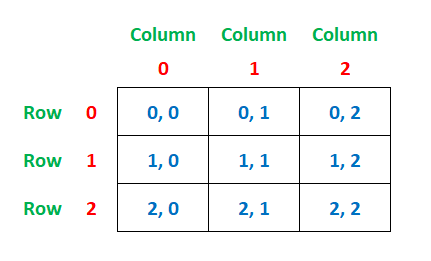In the above image, you can see that we have created a 2D Array having 3 rows and 3 columns. We can call the above array as a 3x3 Matrix.

Note: A 2D array is used to store data in the form of a table.

## Declaration and Initialization of a Two Dimensional Array in C++

In C++ programming a two dimensional array can be declared and initialized in several ways. Let’s see the different ways of initializing a 2D array.

#### Example 1: (with row and column size mentioned)

``int a= {{1,2,3}, {4,5,6}, {7,8,9}};``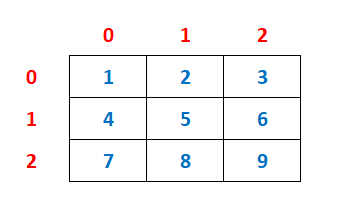#### Example 2: (with only column size mentioned)

``int a[]= {{15,27,36}, {41,52,64}, {79,87,93}};``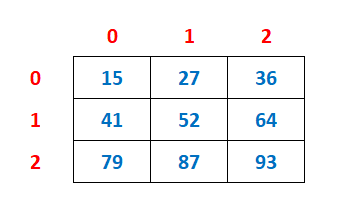#### Example 3: (1st cell contains the value 5 and, rest of the cells 0)

``int a= {{5},{0},{0}};``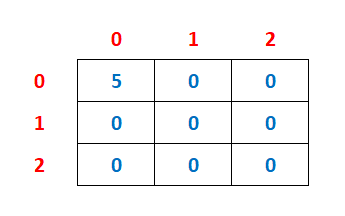#### Example 4: (1st cell of each row contains the value 5 and, rest of the cells 0)

``int a= {{5},{5},{5}};``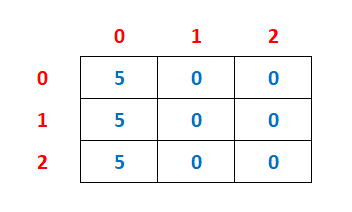#### Example 5: (All cell contains the value 0)

``int a= {{0},{0},{0}};``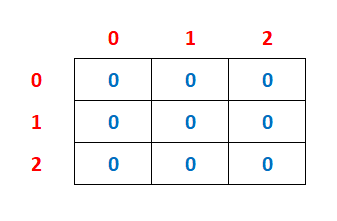## Store Numbers in a Two Dimensional Array

To store the number in each cell of the 2D array we can use the following syntax.

``array_name[row_index][column_index]=value;``

#### Example

``````a=15;
a=27;
a=36;
a=41;
a=52;
a=64;
a=79;
a=87;
a=93;
``````

## Access Numbers in a Two Dimensional Array

We can access any number stored in a 2D array using the following syntax.

``array_name[row_index][column_index]``

#### Example

``````cout<<a<<" "<<a<<" "<<a<<endl;
cout<<a<<" "<<a<<" "<<a<<endl;
cout<<a<<" "<<a<<" "<<a<<endl;
``````

#### Output

```15 27 36
41 52 64
79 87 93
```

## Store and Access the Numbers in a 2D Array using Loops

We can also store as well as access the numbers in a 2D array using either for, while or do while loop. Let's see a few examples.

#### Example 1

Program to input numbers in a 3x3 Matrix and display the numbers in a table format.

``````#include <iostream>
#include <conio.h>

using namespace std;

int main()
{
int a, r,c;

cout<<"Enter 9 numbers\n";
for(r=0; r<3; r++)
{
for(c=0; c<3; c++)
{
cin>>a[r][c];
}
}

cout<<"\nOutput\n";
for(r=0; r<3; r++)			// this loop is for row
{
for(c=0; c<3; c++)		// this loop will print 3 numbers in each row
{
cout<<a[r][c]<<" ";
}
cout<<endl;		// break the line after printing the numbers in a row
}
return 0;
}``````

#### Output

```Enter 9 numbers
84
36
41
98
34
57
20
31
24

Output
84 36 41
98 34 57
20 31 24```

Here, you can see that we have run a nested for loop to store the user's input in a 3x3 matrix. After that we have run another nested for loop to access each number from the 2D array and print on the screen in a table format.

#### Example 2

Program to input numbers in a 3x3 Matrix and print only even numbers if present in the matrix.

``````#include <iostream>
#include <conio.h>

using namespace std;

int main()
{
int a, r,c;

cout<<"Enter 9 numbers\n";
for(r=0; r<3; r++)
{
for(c=0; c<3; c++)
{
cin>>a[r][c];
}
}

cout<<"\nEven Numbers\n";
for(r=0; r<3; r++)
{
for(c=0; c<3; c++)
{
if(a[r][c]%2==0)
{
cout<<a[r][c]<<" ";
}
}
}
return 0;
}``````

#### Output

```Enter 9 numbers
84
36
41
98
34
57
20
31
24

Even Numbers
84 36 98 34 20 24 ```

Here, you can see that we have entered numbers in a 3x3 matrix using nested for loop. After that we have run another nested for loop to fetch the numbers from the 2D array and print only those numbers which are divisible by 2.